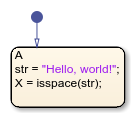# isspace

Determine which characters are space characters

## Syntax

``tf = isspace(A)``

## Description

example

````tf = isspace(A)` returns a logical array `tf`. If `A` is a string, then the elements of `tf` are logical `1` (`true`) where corresponding characters in `A` are space characters, and logical `0` (`false`) elsewhere.```

Note

The operator `isspace` is supported only in Stateflow® charts that use MATLAB® as the action language.

## Examples

expand all

```h = "Hello, world!" x = isspace(h)```## Version History

Introduced in R2021b